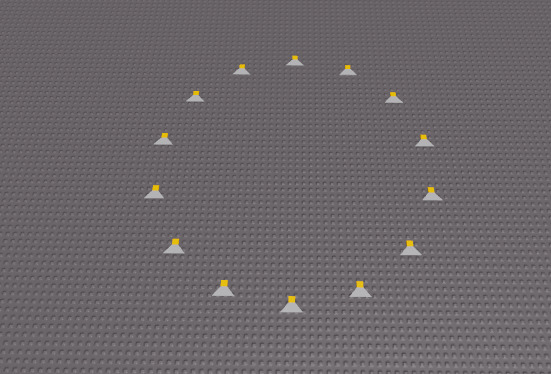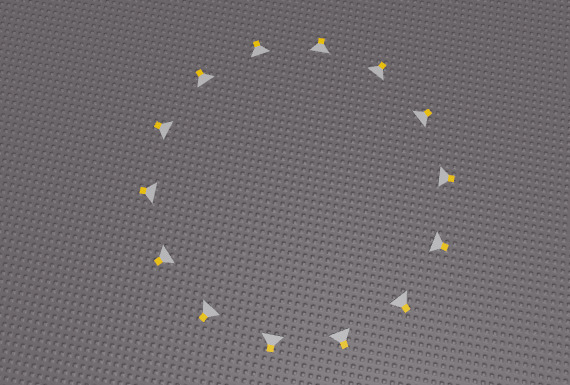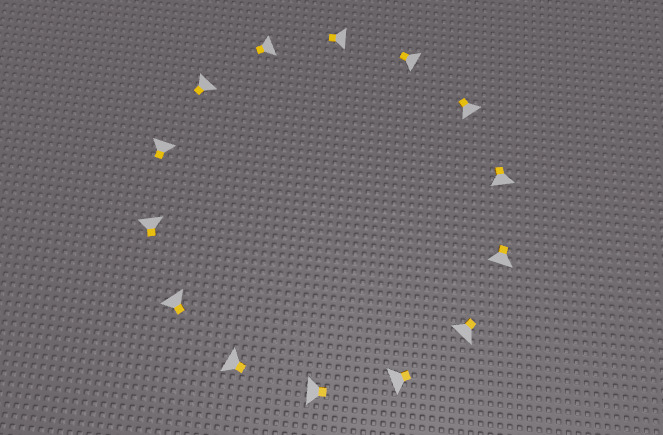# Struggling with a circle made out of wedges

Issue:My end goal is to make a circle out of this shape I made, I got the circle part however cannot seem to figure out the math to make the yellow part face outward of the circle.

End goal example:Current Code:

``````local radius = 20
local Size = 5
local number_of_parts = radius / Size + 10
local circle = math.pi * 2

for i=1, number_of_parts do

local angle = circle / number_of_parts * i
local x_pos = math.cos(angle) * radius
local y_pos = math.sin(angle) * radius

local part = game.Workspace.Wedge:Clone()
part.Parent = game.Workspace

end
``````

Found this and edited it off of another forum post, however, I could not seem to find a post detailing my issue.

Any help would be appreciated thanks!

Try out

``````part:SetPrimaryPartCFrame(CFrame.new(Vector3.new(x_pos, 0, y_pos), Vector3.new()))
``````

This is the result:Somone said to use the center of the circle, however, I don’t know how to use that formula in lua.

My code generates a CFrame at the point you want to place the wedge looking at the center of the circle, since you seem to have the center to be 0, 0, 0, I just used Vector3.new(), You generate it like this CFrame.new(position, lookAt)
Now we just need to rotate these parts 90 degrees so they look where we want them!
Try this

``````part:SetPrimaryPartCFrame(CFrame.new(Vector3.new(x_pos, 0, y_pos), Vector3.new()) * CFrame.fromEulerAnglesXYZ(0, math.pi / 2, 0))
``````

Or

``````part:SetPrimaryPartCFrame(CFrame.new(Vector3.new(x_pos, 0, y_pos), Vector3.new()) * CFrame.fromEulerAnglesXYZ(0, -math.pi / 2, 0))
``````

Thank you for help, I felt like I should have listened more in Geometry.

1 Like